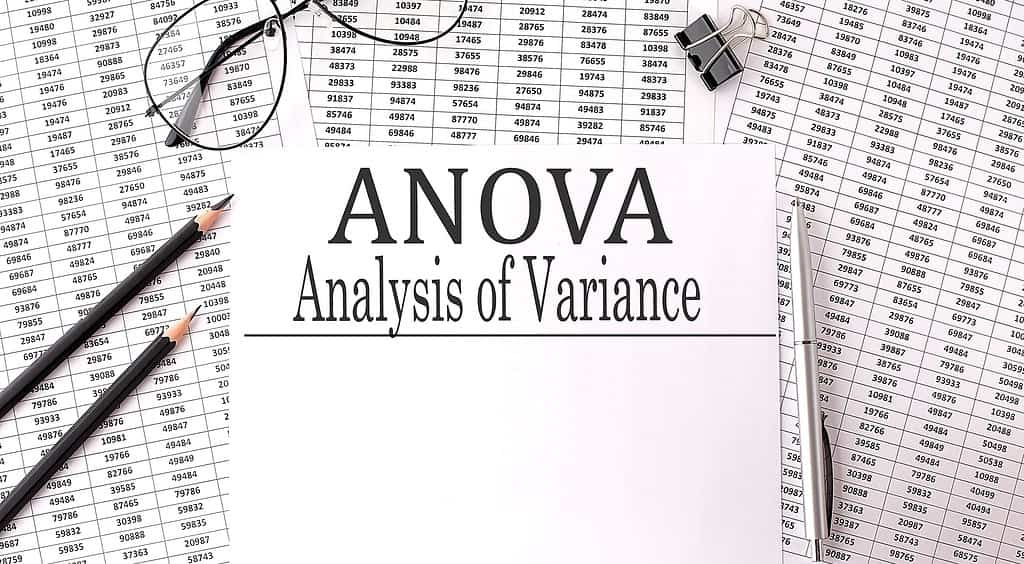## The Importance of Fisher’s (1-way ANOVA) in Statistical Analysis

Updated:

Fisher’s 1-way ANOVA is a classic analysis of variance utilized in statistics to determine if there are statistical differences between the means of two or more unrelated groups.## ANOVA: A Simple Guide to Comparing Multiple Group Means

Updated:

ANOVA is a powerful statistical method for comparing several samples (3 or more) to each other to see if the populations that the means came from are statistically different.

## Understanding the Mood’s Median Test: An Overview

Updated:

Your data has failed the assumption of normality needed for a parametric test of hypotheses. If you have two or more groups you want to analyze, what do you do? Maybe the Mood’s Median Test will help.## The F test: An Essential Tool for Data Analysis and Hypothesis Testing

Updated:

In many cases, you want to compare the means of samples. But, comparing variances can be just as important. The F test is the statistical tool you would use for that.## Understanding the basics of ANOVA and Dunnett’s 1-way ANOVA

Updated:

If you are curious as to whether the means of greater than 2 groups are statistically different or not you could use a 1-way ANOVA. But, if you have multiple treatment groups and want to compare the means to a control group, then you can use Dunnett’s 1-way ANOVA.  ANOVA stands for Analysis of Variance. […]## How To Compare Data Sets – ANOVA

Published:

In 1920, Sir Ronald A. Fisher invented a statistical way to compare data sets. Fisher called his method the analysis of variance, which was later dubbed an ANOVA. This method eventually evolved into Six Sigma data set comparisons. The F ratio is the probability information produced by an ANOVA. It was named for Fisher. The […]## Help for Practitioners Trying to Understand ANOVA Table

Published:

The analysis of variance (ANOVA) procedure is conducted during the Analyze phase of a Six Sigma project. Assessing results from an ANOVA table can present a challenge making it difficult to understand precisely what conclusions to draw. However, there is an easy way for Master Black Belts to explain to their charges the ANOVA procedure. […]## Using ANOVA to Find Differences in Population Means

Published:

Three methods used to dissolve a powder in water are compared by the time (in minutes) it takes until the powder is fully dissolved. The results are summarized in the following table: It is thought that the population means of the three methods m1, m2 and m3 are not all equal (i.e., at least one m […]